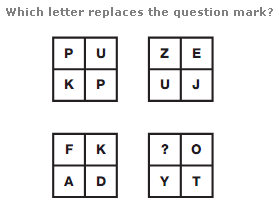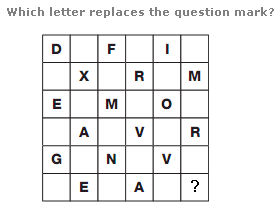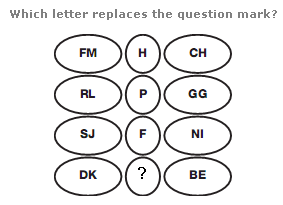# Puzzles - Missing letters puzzles

### Exercise :: Missing letters puzzlesAnswer : Z Explanation : Read the diagram as a whole grid of 4x4 squares. Starting on the bottom left square, move clockwise around the outside of the whole square, with letters written in sequence, skipping 4 letters at a time. Repeat this instruction for the small inner square.Answer : X Explanation : Start at the top left and move in alternate boxes from left to right, then down one row and to the left, in a snakes and ladders pattern. Letters advance through the alphabet in steps of 2, 3, 4...8, before repeating this pattern.Answer : H Explanation : In each row, the numerical value of the central letter equals the difference between the sum of the numerical values of the two left hand letters and the sum of the numerical values of the two right hand letters.